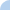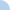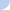# Regression lines and correlation r (Java type): session regression lines and correlation r (java type)

Use the following: The least-squares regression line based on 43 observations of female white rats is y = 95 + 32x, where x is the weeks after birth and y is weight in grams. The correlation r is 0.78.First name Last nameDavid Farnsworth

 This activity was created by a Quia Web subscriber. Learn more about QuiaCreate your own activities